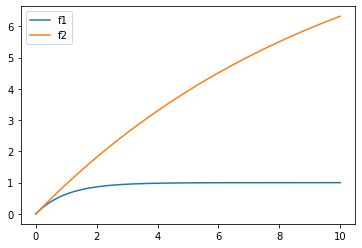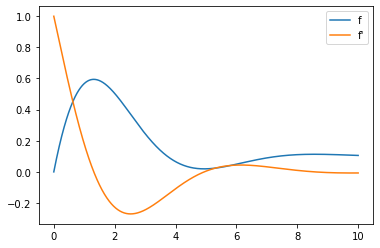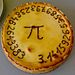### Differential equation in python

###### Sat 04 April 2020
Second order differential equation (Photo credit: Wikipedia)

In python, differential equations can be numerically solved thanks to scipy . Is usage is not as intuitive as I expected.

## Simple equation

Let's start small. The first equation will be really simple:

\begin{equation*} \frac{\partial{f}}{\partial{t}} = a \times f + b \end{equation*}

The scipy ode module asks for equations written that way ($$\frac{\partial{f}}{\partial{t}}=fun(...)$$)

def first_fun(y, t, a=-1, b=1):
return a * y + b


This function takes as input:

• $$y$$ : the value of $$f(t)$$
• $$t$$ : the variable use by 'scipy ode'
• other parameters

Now let's define the variable t for which wee want a value:

import numpy as np

t = np.linspace(0, 100, 1000)


Now let's ask scipy for a solution:

from scipy.integrate import odeint

init_value = 0
solution = odeint(first_fun, init_value, t)


And let's plot it. As I am a lazy person, I'll let pandas plot it:

import pandas as pd

pd.DataFrame(solution, index=t).plot()## Multiple equations

Let's complicate things (a bit). The function can be multidimensional. This way, we can have multiple functions in our system of differential equations, either independent or not. In either way, we will build a function returning

\begin{equation*} \begin{bmatrix} \frac{\partial{f_1}}{\partial{t}} \\ \vdots\\ \frac{\partial{f_n}}{\partial{t}} \end{bmatrix} \end{equation*}

and taking as input

\begin{equation*} \begin{bmatrix} f_1 \\ \vdots\\ f_n \end{bmatrix} \end{equation*}
def indep_fun(y, t, a1=-1, a2=.1, b1=1, b2=1):
f1, f2 = y
return [
a1 * f1 + b1, # \frac{\partial{f1}}{\partial{t}}
a2 * f2 + b2, # \frac{\partial{f2}}{\partial{t}}
]


And let's solve and plot it:

int_value = [0, 0] # [f1(0), f2(0)]
solution = odeint(first_fun, init_value, t)
pd.DataFrame(solution, index=t, columns=("f1", "f2")).plot()## Second order differential equation

For that step, we need to take indirect routes. The basic principle is to use multiple equations, the second one being the derivative of the first one.

Let's say our equation is:

\begin{equation*} \frac{\partial^2 {f}}{\partial{t}^2} = a\times\frac{\partial{f}}{\partial{t}} + b\times f + c \end{equation*}

The input of our python function will be:

\begin{equation*} \begin{bmatrix} {f} \\ \frac{\partial{f}}{\partial{t}} \end{bmatrix} \end{equation*}

and the output:

\begin{equation*} \begin{bmatrix} \frac{\partial{f}}{\partial{t}}\\ \frac{\partial^2 {f}}{\partial{t}^2} \end{bmatrix} \end{equation*}

Thus, the code is:

def second_order(y, t, a=-1, b=-1, c=.1):
f, df = y
return [
df, # \partial{f}/\partial{t}
a * df + b * f + c, # \partial^2 {f}/\partial{t}^2
]

init_value = [
0, # f(0)
1, # f'(0)
]
solution = odeint(first_fun, init_value, t)
pd.DataFrame(solution, index=t, columns=("f", "f'")).plot()notebook to generate graphs

Category: maths Tagged: python maths equation

### Zombie propagation

###### Sat 21 March 2020
Zombie favorite food warning (Photo credit: wikipedia)

I recently read a paper  trying to model a disease propagation. I wanted to play with this model.

## The model

The model is know as "SIR" as it divide the population into 3 groups:

• S: suceptible to become a zombie
• I: infected …

Category: maths Tagged: python maths zombie

### Happy pi day

###### Sat 14 March 2015Pi Pie (Photo credit: Wikipedia)

On this Pi day , I would like to enumerate all uses of pi I know. There are more ( listed here ):

Category: useless Tagged: Pi Day maths useless

### Puzzle

###### Mon 20 January 2014Famous 3D puzzle (Photo credit: Wikipedia)

The question is: what is a puzzle? The answer I prefer is: a riddle whose solution is hard to find but easy to verify. In computer science, it’s used to provide proof-of-work and usually implemented through cryptographic mechanisms. I found versions of cryptographic …

### Music reflection: timeline and publications

###### Sat 18 January 2014

[caption id="" align="alignright" width="350"]sound research material (Photo credit: Wikipedia)[/caption]

### music physics: rhythms

###### Fri 03 January 2014

[caption id="" align="alignright" width="300"]Natural rhythms generator[/caption]

I'm still interesseted in music (de)composition. In this post, I want to focus on rhythm, i.e. only when a note is played and for how many time.

## notation

I will use the usual notation is sheets.

## note duration …

Category: maths Tagged: bar beat duration note rhythm Typesetting music maths